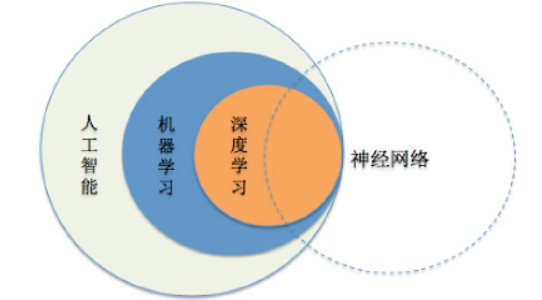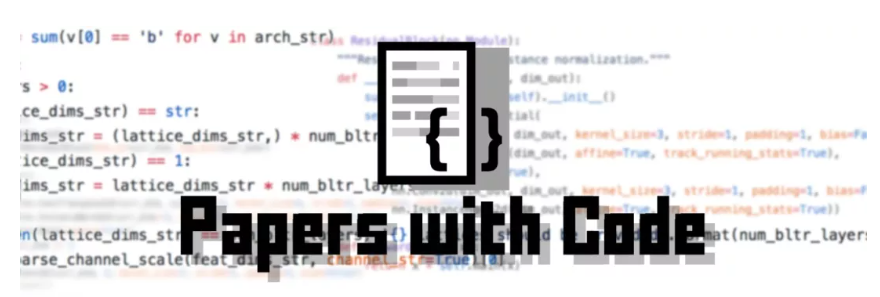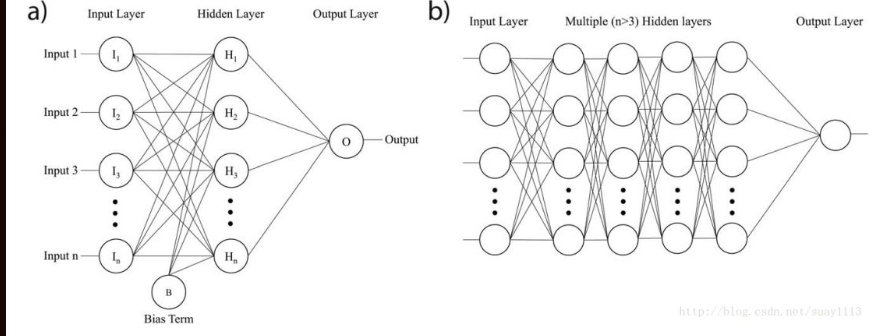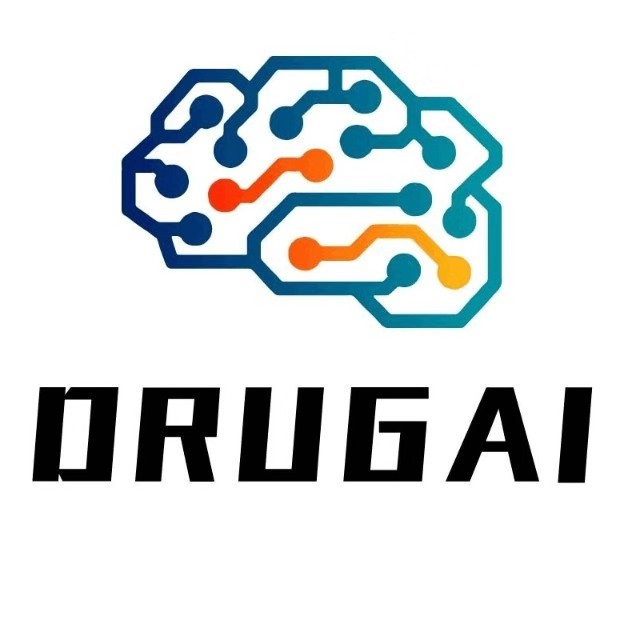# 准确率98%的深度学习交通标志识别是如何做到的？

+关注继续查看## 项目设置

• 图像为32（宽）×32（高）×3（RGB彩色通道）
• 训练集由34799张图片组成
• 验证集由4410个图像组成
• 测试集由12630个图像组成
• 共有43个种类（例如限速20公里/小时、禁止进入、颠簸路等等）

### 图像及其分布## 预处理步骤

### 灰度化### 归一化## 模型的架构mc_3x3 = ModelConfig(EdLeNet, "EdLeNet_Norm_Grayscale_3x3_Dropout_0.50", [32, 32, 1], [3, 32, 3], [120, 84], n_classes, [0.75, 0.5])
mc_5x5 = ModelConfig(EdLeNet, "EdLeNet_Norm_Grayscale_5x5_Dropout_0.50", [32, 32, 1], [5, 32, 2], [120, 84], n_classes, [0.75, 0.5])

me_g_norm_drpt_0_50_3x3 = ModelExecutor(mc_3x3)
me_g_norm_drpt_0_50_5x5 = ModelExecutor(mc_5x5)

ModelConfig包含了模型的相关信息，比如：

• 模型的函数（例如：EdLeNet
• 模型的名称
• 输入的格式（例如：灰度等级为[32,32,1]），
• 卷积层的配置[过滤器大小、起始深度、层数]，
• 完全连接层的大小（例如：[120,84]）
• 种类的数量
• dropout（丢弃）百分比值[p-conv，p-fc]

ModelExecutor负责_训练_、_评估_、_预测_，以及生成_激活_映射的可视化效果。

self.graph = tf.Graph()
with self.graph.as_default() as g:
with g.name_scope( self.model_config.name ) as scope:

...

with tf.Session(graph = self.graph) as sess:## Dropout（丢弃）算法

p-conv: probability of keep weight in convolutional layer
p-fc: probability of keeping weight in fully connected layer

p-conv >= p-fc

Training EdLeNet_Norm_Grayscale_3x3_Dropout_0.50 [epochs=100, batch_size=512]...

    total=5.222s | train: time=3.139s, loss=3.4993, acc=0.1047 | val: time=2.083s, loss=3.5613, acc=0.1007
    total=5.190s | train: time=3.122s, loss=0.2589, acc=0.9360 | val: time=2.067s, loss=0.3260, acc=0.8973
...
    total=5.193s | train: time=3.120s, loss=0.0006, acc=0.9999 | val: time=2.074s, loss=0.0747, acc=0.9841
    total=5.191s | train: time=3.123s, loss=0.0004, acc=1.0000 | val: time=2.068s, loss=0.0849, acc=0.9832
Model ./models/EdLeNet_Norm_Grayscale_3x3_Dropout_0.50.chkpt saved
[EdLeNet_Norm_Grayscale_3x3_Dropout_0.50 - Test Set]    time=0.686s, loss=0.1119, acc=0.9755## 直方图均衡化

Training EdLeNet_Grayscale_CLAHE_Norm_Take-2_3x3_Dropout_0.50 [epochs=500, batch_size=512]...
    total=5.194s | train: time=3.137s, loss=3.6254, acc=0.0662 | val: time=2.058s, loss=3.6405, acc=0.0655
    total=5.155s | train: time=3.115s, loss=0.8645, acc=0.7121 | val: time=2.040s, loss=0.9159, acc=0.6819
...
    total=5.149s | train: time=3.106s, loss=0.0009, acc=0.9998 | val: time=2.042s, loss=0.0355, acc=0.9884
    total=5.148s | train: time=3.106s, loss=0.0007, acc=0.9998 | val: time=2.042s, loss=0.0390, acc=0.9884
    total=5.148s | train: time=3.104s, loss=0.0006, acc=0.9999 | val: time=2.044s, loss=0.0420, acc=0.9862
Model ./models/EdLeNet_Grayscale_CLAHE_Norm_Take-2_3x3_Dropout_0.50.chkpt saved
[EdLeNet_Grayscale_CLAHE_Norm_Take-2_3x3_Dropout_0.50 - Test Set]    time=0.675s, loss=0.0890, acc=0.9775

## 数据增强

1. 扩展数据集，并在不同的照明条件和方向上提供其他图片
2. 提高模型的通用性
3. 提高测试和验证的准确性，特别是对失真的图像

def augment_imgs(imgs, p):
"""
Performs a set of augmentations with with a probability p
"""
augs =  iaa.SomeOf((2, 4),
[
iaa.Crop(px=(0, 4)), # crop images from each side by 0 to 4px (randomly chosen)
iaa.Affine(scale={"x": (0.8, 1.2), "y": (0.8, 1.2)}),
iaa.Affine(translate_percent={"x": (-0.2, 0.2), "y": (-0.2, 0.2)}),
iaa.Affine(rotate=(-45, 45)), # rotate by -45 to +45 degrees)
iaa.Affine(shear=(-10, 10)) # shear by -10 to +10 degrees
])

seq = iaa.Sequential([iaa.Sometimes(p, augs)])

return seq.augment_images(imgs)[EdLeNet] Building neural network [conv layers=3, conv filter size=3, conv start depth=32, fc layers=2]
Training EdLeNet_Augs_Grayscale_CLAHE_Norm_Take4_Bis_3x3_Dropout_0.50 [epochs=2000, batch_size=512]...

    total=5.824s | train: time=3.594s, loss=3.6283, acc=0.0797 | val: time=2.231s, loss=3.6463, acc=0.0687
...
    total=5.627s | train: time=3.408s, loss=0.0525, acc=0.9870 | val: time=2.219s, loss=0.0315, acc=0.9914
    total=5.627s | train: time=3.409s, loss=0.0530, acc=0.9862 | val: time=2.218s, loss=0.0309, acc=0.9902
    total=5.628s | train: time=3.412s, loss=0.0521, acc=0.9869 | val: time=2.216s, loss=0.0302, acc=0.9900
    total=5.632s | train: time=3.415s, loss=0.0521, acc=0.9869 | val: time=2.217s, loss=0.0311, acc=0.9902
Model ./models/EdLeNet_Augs_Grayscale_CLAHE_Norm_Take4_Bis_3x3_Dropout_0.50.chkpt saved

[EdLeNet_Augs_Grayscale_CLAHE_Norm_Take4_Bis_3x3_Dropout_0.50 - Test Set]    time=0.678s, loss=0.0842, acc=0.9786

### 这是迄今为止最好的结果!!!## 结论60 010 年深度学习顶级论文和代码精选，请务必收藏！
10 年深度学习顶级论文和代码精选，请务必收藏！
77 091 0laravel中短信发送验证码的实现方法

3235 020篇顶级深度学习论文（附链接）
4638 02852 0984 01784 0LVM学习之LVM基础
3286 0【学生来信】亲爱的老师： 弟子发现一个重大问题，上课听得明白，上机时却什么也想不起来，对一切都陌生，您说的什么都让我给忘了，应该是听课效率低吧，究竟是什么情况？我晕啊！老师可能说，这需要一个过程，但是，我认为应该时刻调整自己，尽快找到适合自己的方式方法，让自己快速熟悉起来，不要那么陌生，更不要老忘东西，这是学习的大忌吧。老师若有偏方可医疗我的疑难杂症，不防支给弟子一式半招，小徒定当感激涕泠！【
839 0
【方向】

706

5